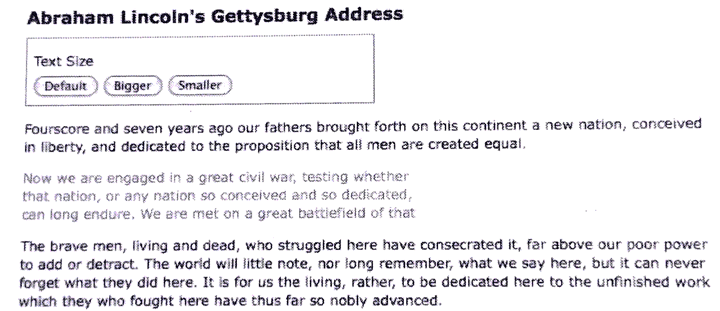kaldi数据准备

kaldi开发技术服务

Java 安卓移动开发
VC++
C#软件

Q Q：396806883

# 简介

## 实例

\$( document ). ready ( function( ) {

\$( 'p' ). eq( 1 ). hide( );

\$( 'a. more' ). click( function ( ){

\$( 'p' ). eq( 1 ). show( 'slow' );

\$( this ). hide( );

return false;

});

});### 1.淡入和淡出

\$( document ). ready ( function( ) {

\$( 'p' ). eq( 1 ). hide( );

\$( 'a. more' ). click( function ( ){

\$( 'p' ). eq( 1 ). fadeIn( 'slow' );

\$( this ). hide( );

return false;

});

});

### 2.滑上和滑下

\$( document ). ready ( function( ) {

\$( 'p' ). eq( 1 ). hide( );

\$( 'a. more' ). click( function ( ){

\$( 'p' ). eq( 1 ). slideDown( 'slow' );

\$( this ). hide( );

return false;

});

});

### 3.复合效果

\$( document ). ready ( function( ) {

var \$firstPara=\$( 'p' ). eq( 1 );

\$firstPara. hide( );

\$( 'a. more' ). click( function ( ){

if (\$firstPara. is( ':hidden' )){

\$( this ). text( 'read less' );

}else{

\$( this ). text( 'read more' );

}

return false;

});

});

\$( document ). ready ( function( ) {

var \$firstPara=\$( 'p' ). eq( 1 );

\$firstPara. hide( );

\$( 'a. more' ). click( function ( ){

\$firstPara. slideToggle( 'slow' );

}else{

}

return false;

});

});